# anova1

One-way analysis of variance

## Syntax

``p = anova1(y)``
``p = anova1(y,group)``
``p = anova1(y,group,displayopt)``
``````[p,tbl] = anova1(___)``````
``````[p,tbl,stats] = anova1(___)``````

## Description

example

````p = anova1(y)` performs one-way ANOVA for the sample data `y` and returns the p-value. `anova1` treats each column of `y` as a separate group. The function tests the hypothesis that the samples in the columns of `y` are drawn from populations with the same mean against the alternative hypothesis that the population means are not all the same. The function also displays the box plot for each group in `y` and the standard ANOVA table (`tbl`).```
````p = anova1(y,group)` performs one-way ANOVA for the sample data `y`, grouped by `group`.```

example

````p = anova1(y,group,displayopt)` enables the ANOVA table and box plot displays when `displayopt` is `'on'` (default) and suppresses the displays when `displayopt` is `'off'`.```

example

``````[p,tbl] = anova1(___)``` returns the ANOVA table (including column and row labels) in the cell array `tbl` using any of the input argument combinations in the previous syntaxes. To copy a text version of the ANOVA table to the clipboard, select Edit > Copy Text from the ANOVA table figure.```

example

``````[p,tbl,stats] = anova1(___)``` returns a structure, `stats`, which you can use to perform a multiple comparison test. A multiple comparison test enables you to determine which pairs of group means are significantly different. To perform this test, use `multcompare`, providing the `stats` structure as an input argument.```

## Examples

collapse all

Create sample data matrix `y` with columns that are constants, plus random normal disturbances with mean 0 and standard deviation 1.

```y = meshgrid(1:5); rng default; % For reproducibility y = y + normrnd(0,1,5,5)```
```y = 5×5 1.5377 0.6923 1.6501 3.7950 5.6715 2.8339 1.5664 6.0349 3.8759 3.7925 -1.2588 2.3426 3.7254 5.4897 5.7172 1.8622 5.5784 2.9369 5.4090 6.6302 1.3188 4.7694 3.7147 5.4172 5.4889 ```

Perform one-way ANOVA.

`p = anova1(y)`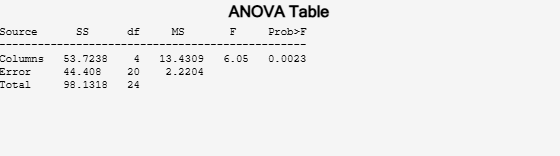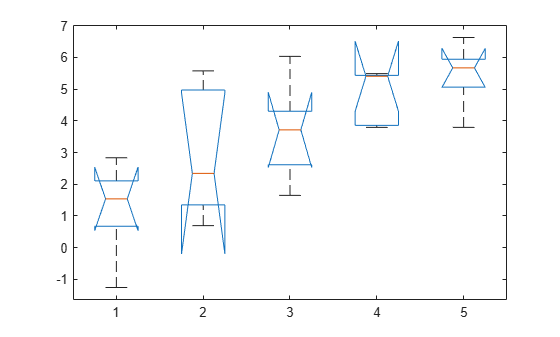```p = 0.0023 ```

The ANOVA table shows the between-groups variation (`Columns`) and within-groups variation (`Error`). `SS` is the sum of squares, and `df` is the degrees of freedom. The total degrees of freedom is total number of observations minus one, which is 25 - 1 = 24. The between-groups degrees of freedom is number of groups minus one, which is 5 - 1 = 4. The within-groups degrees of freedom is total degrees of freedom minus the between groups degrees of freedom, which is 24 - 4 = 20.

`MS` is the mean squared error, which is `SS/df` for each source of variation. The F-statistic is the ratio of the mean squared errors (13.4309/2.2204). The p-value is the probability that the test statistic can take a value greater than the value of the computed test statistic, i.e., P(F > 6.05). The small p-value of 0.0023 indicates that differences between column means are significant.

Input the sample data.

```strength = [82 86 79 83 84 85 86 87 74 82 ... 78 75 76 77 79 79 77 78 82 79]; alloy = {'st','st','st','st','st','st','st','st',... 'al1','al1','al1','al1','al1','al1',... 'al2','al2','al2','al2','al2','al2'};```

The data are from a study of the strength of structural beams in Hogg (1987). The vector strength measures deflections of beams in thousandths of an inch under 3000 pounds of force. The vector alloy identifies each beam as steel (`'st'`), alloy 1 (`'al1'`), or alloy 2 (`'al2'`). Although alloy is sorted in this example, grouping variables do not need to be sorted.

Test the null hypothesis that the steel beams are equal in strength to the beams made of the two more expensive alloys. Turn the figure display off and return the ANOVA results in a cell array.

`[p,tbl] = anova1(strength,alloy,'off')`
```p = 1.5264e-04 ```
```tbl=4×6 cell array {'Source'} {'SS' } {'df'} {'MS' } {'F' } {'Prob>F' } {'Groups'} {[184.8000]} {[ 2]} {[ 92.4000]} {[ 15.4000]} {[1.5264e-04]} {'Error' } {[102.0000]} {} {[ 6.0000]} {0x0 double} {0x0 double } {'Total' } {[286.8000]} {} {0x0 double} {0x0 double} {0x0 double } ```

The total degrees of freedom is total number of observations minus one, which is $20-1=19$. The between-groups degrees of freedom is number of groups minus one, which is $3-1=2$. The within-groups degrees of freedom is total degrees of freedom minus the between groups degrees of freedom, which is $19-2=17$.

`MS` is the mean squared error, which is `SS/df` for each source of variation. The F-statistic is the ratio of the mean squared errors. The p-value is the probability that the test statistic can take a value greater than or equal to the value of the test statistic. The p-value of 1.5264e-04 suggests rejection of the null hypothesis.

You can retrieve the values in the ANOVA table by indexing into the cell array. Save the F-statistic value and the p-value in the new variables `Fstat` and `pvalue`.

`Fstat = tbl{2,5}`
```Fstat = 15.4000 ```
`pvalue = tbl{2,6}`
```pvalue = 1.5264e-04 ```

Input the sample data.

```strength = [82 86 79 83 84 85 86 87 74 82 ... 78 75 76 77 79 79 77 78 82 79]; alloy = {'st','st','st','st','st','st','st','st',... 'al1','al1','al1','al1','al1','al1',... 'al2','al2','al2','al2','al2','al2'};```

The data are from a study of the strength of structural beams in Hogg (1987). The vector strength measures deflections of beams in thousandths of an inch under 3000 pounds of force. The vector alloy identifies each beam as steel (`st`), alloy 1 (`al1`), or alloy 2 (`al2`). Although alloy is sorted in this example, grouping variables do not need to be sorted.

Perform one-way ANOVA using `anova1`. Return the structure `stats`, which contains the statistics `multcompare` needs for performing Multiple Comparisons.

`[~,~,stats] = anova1(strength,alloy);`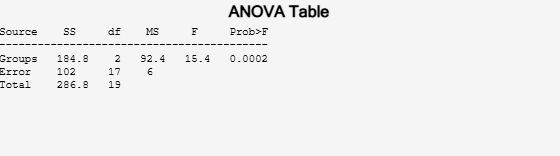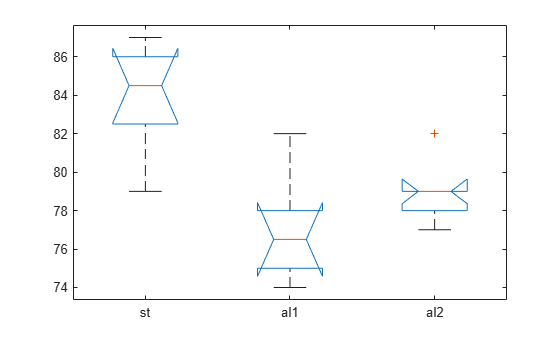The small p-value of 0.0002 suggests that the strength of the beams is not the same.

Perform a multiple comparison of the mean strength of the beams.

`[c,~,~,gnames] = multcompare(stats);`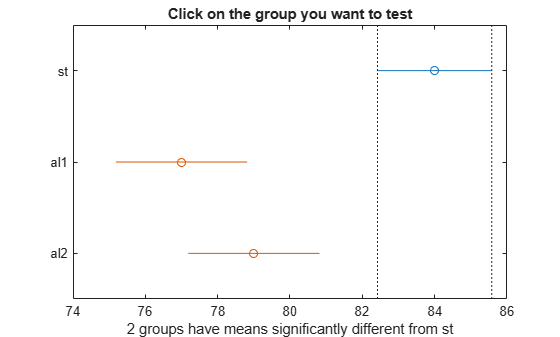In the figure, the blue bar represents the comparison interval for mean material strength for steel. The red bars represent the comparison intervals for the mean material strength for alloy 1 and alloy 2. Neither of the red bars overlaps with the blue bar, which indicates that the mean material strength for steel is significantly different from that of alloy 1 and alloy 2. You can confirm the significant difference by clicking the bars that represent alloy 1 and 2.

Display the multiple comparison results and the corresponding group names in a table.

```tbl = array2table(c,"VariableNames", ... ["Group A","Group B","Lower Limit","A-B","Upper Limit","P-value"]); tbl.("Group A") = gnames(tbl.("Group A")); tbl.("Group B") = gnames(tbl.("Group B"))```
```tbl=3×6 table Group A Group B Lower Limit A-B Upper Limit P-value _______ _______ ___________ ___ ___________ __________ {'st' } {'al1'} 3.6064 7 10.394 0.00016831 {'st' } {'al2'} 1.6064 5 8.3936 0.0040464 {'al1'} {'al2'} -5.628 -2 1.628 0.35601 ```

The first two columns show the pair of groups that are compared. The fourth column shows the difference between the estimated group means. The third and fifth columns show the lower and upper limits for the 95% confidence intervals of the true difference of means. The sixth column shows the p-value for a hypothesis that the true difference of means for the corresponding groups is equal to zero.

The first two rows show that both comparisons involving the first group (steel) have confidence intervals that do not include zero. Because the corresponding p-values (1.6831e-04 and 0.0040, respectively) are small, those differences are significant.

The third row shows that the differences in strength between the two alloys is not significant. A 95% confidence interval for the difference is [-5.6,1.6], so you cannot reject the hypothesis that the true difference is zero. The corresponding p-value of 0.3560 in the sixth column confirms this result.

## Input Arguments

collapse all

Sample data, specified as a vector or matrix.

• If `y` is a vector, you must specify the `group` input argument. Each element in `group` represents a group name of the corresponding element in `y`. The `anova1` function treats the `y` values corresponding to the same value of `group` as part of the same group. Use this design when groups have different numbers of elements (unbalanced ANOVA).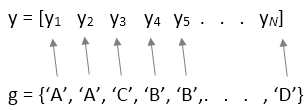• If `y` is a matrix and you do not specify `group`, then `anova1` treats each column of `y` as a separate group. In this design, the function evaluates whether the population means of the columns are equal. Use this design when each group has the same number of elements (balanced ANOVA).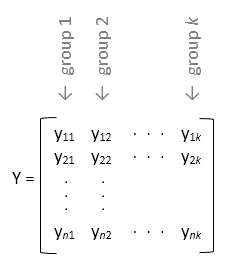• If `y` is a matrix and you specify `group`, then each element in `group` represents a group name for the corresponding column in `y`. The `anova1` function treats the columns that have the same group name as part of the same group.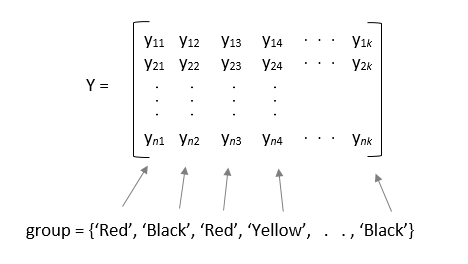Note

`anova1` ignores any `NaN` values in `y`. Also, if `group` contains empty or `NaN` values, `anova1` ignores the corresponding observations in `y`. The `anova1` function performs balanced ANOVA if each group has the same number of observations after the function disregards empty or `NaN` values. Otherwise, `anova1` performs unbalanced ANOVA.

Data Types: `single` | `double`

Grouping variable containing group names, specified as a numeric vector, logical vector, categorical vector, character array, string array, or cell array of character vectors.

• If `y` is a vector, then each element in `group` represents a group name of the corresponding element in `y`. The `anova1` function treats the `y` values corresponding to the same value of `group` as part of the same group.N is the total number of observations.

• If `y` is a matrix, then each element in `group` represents a group name for the corresponding column in `y`. The `anova1` function treats the columns of `y` that have the same group name as part of the same group.If you do not want to specify group names for the matrix sample data `y`, enter an empty array (`[]`) or omit this argument. In this case, `anova1` treats each column of `y` as a separate group.

If `group` contains empty or `NaN` values, `anova1` ignores the corresponding observations in `y`.

Example: `'group',[1,2,1,3,1,...,3,1]` when `y` is a vector with observations categorized into groups 1, 2, and 3

Example: `'group',{'white','red','white','black','red'}` when `y` is a matrix with five columns categorized into groups red, white, and black

Data Types: `single` | `double` | `logical` | `categorical` | `char` | `string` | `cell`

Indicator to display the ANOVA table and box plot, specified as `'on'` or `'off'`. When `displayopt` is `'off'`, `anova1` returns the output arguments, only. It does not display the standard ANOVA table and box plot.

Example: `p = anova(x,group,'off')`

## Output Arguments

collapse all

p-value for the F-test, returned as a scalar value. p-value is the probability that the F-statistic can take a value larger than the computed test-statistic value. `anova1` tests the null hypothesis that all group means are equal to each other against the alternative hypothesis that at least one group mean is different from the others. The function derives the p-value from the cdf of the F-distribution.

A p-value that is smaller than the significance level indicates that at least one of the sample means is significantly different from the others. Common significance levels are 0.05 or 0.01.

ANOVA table, returned as a cell array. `tbl` has six columns.

ColumnDefinition
`source`The source of the variability.
`SS`The sum of squares due to each source.
`df`The degrees of freedom associated with each source. Suppose N is the total number of observations and k is the number of groups. Then, Nk is the within-groups degrees of freedom (`Error`), k – 1 is the between-groups degrees of freedom (`Columns`), and N – 1 is the total degrees of freedom. N – 1 = (Nk) + (k – 1)
`MS`The mean squares for each source, which is the ratio `SS/df`.
`F`F-statistic, which is the ratio of the mean squares.
`Prob>F`The p-value, which is the probability that the F-statistic can take a value larger than the computed test-statistic value. `anova1` derives this probability from the cdf of F-distribution.

The rows of the ANOVA table show the variability in the data that is divided by the source.

RowDefinition
`Groups`Variability due to the differences among the group means (variability between groups)
`Error`Variability due to the differences between the data in each group and the group mean (variability within groups)
`Total`Total variability

Statistics for multiple comparison tests, returned as a structure with the fields described in this table.

Field nameDefinition
`gnames`Names of the groups
`n`Number of observations in each group
`source`Source of the `stats` output
`means`Estimated values of the means
`df`Error (within-groups) degrees of freedom (Nk, where N is the total number of observations and k is the number of groups)
`s`Square root of the mean squared error

collapse all

### Box Plot

`anova1` returns a box plot of the observations for each group in `y`. Box plots provide a visual comparison of the group location parameters.

On each box, the central mark is the median (2nd quantile, q2) and the edges of the box are the 25th and 75th percentiles (1st and 3rd quantiles, q1 and q3, respectively). The whiskers extend to the most extreme data points that are not considered outliers. The outliers are plotted individually using the `'+'` symbol. The extremes of the whiskers correspond to q3 + 1.5 × (q3q1) and q1 – 1.5 × (q3q1).

Box plots include notches for the comparison of the median values. Two medians are significantly different at the 5% significance level if their intervals, represented by notches, do not overlap. This test is different from the F-test that ANOVA performs; however, large differences in the center lines of the boxes correspond to a large F-statistic value and correspondingly a small p-value. The extremes of the notches correspond to q2 – 1.57(q3q1)/sqrt(n) and q2 + 1.57(q3q1)/sqrt(n), where n is the number of observations without any `NaN` values. In some cases, notches can extend outside the boxes.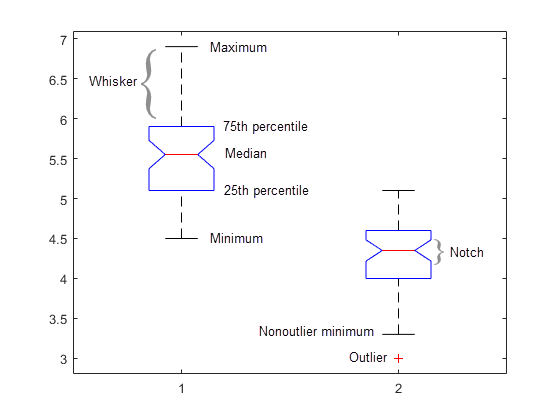For more information about box plots, see `'Whisker'` and `'Notch'` of `boxplot`.

## Alternative Functionality

Instead of using `anova1`, you can create an `anova` object by using the `anova` function. The `anova` function provides these advantages:

• The `anova` function allows you to specify the ANOVA model type, sum of squares type, and factors to treat as categorical. `anova` also supports table predictor and response input arguments.

• In addition to the outputs returned by `anova1`, the properties of the `anova` object contain the following:

• ANOVA model formula

• Fitted ANOVA model coefficients

• Residuals

• Factors and response data

• The `anova` object functions allow you to conduct further analysis after fitting the `anova` object. For example, you can create an interactive plot of multiple comparisons of means for the ANOVA, get the mean response estimates for each value of a factor, and calculate the variance component estimates.

 Hogg, R. V., and J. Ledolter. Engineering Statistics. New York: MacMillan, 1987.